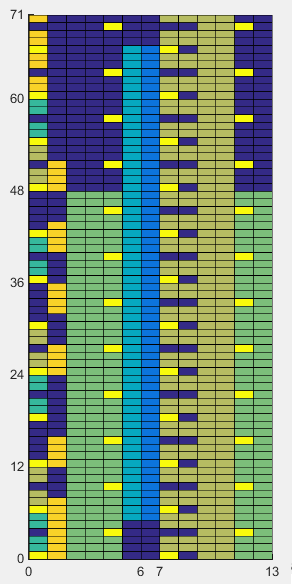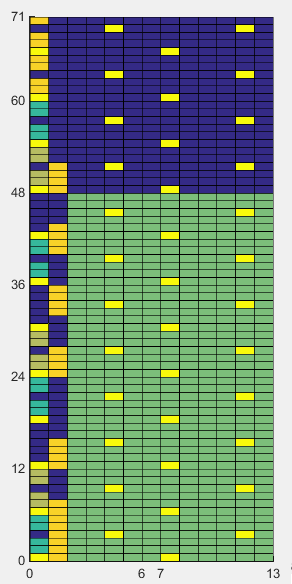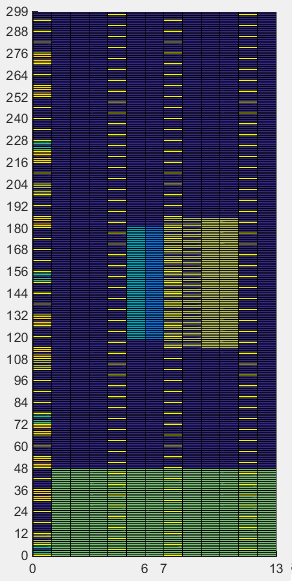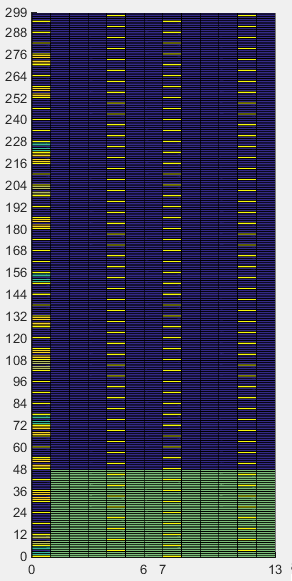MatLab Toolbox - LTE                                      Home : www.sharetechnote.com

PDSCH

If you don't know what PBCH(Physical BroadCasting Channel) is, refer to Physical Layer Channel : Downlink : PDSCH (Physical Downlink Shared Channel) page first. PDSCH Processing would be very complicated one and would require some additional processes that you haven't payed much attention in other channels. I would recommend you to go through all the links mentioned in Physical Layer Channel : Downlink : PDSCH (Physical Downlink Shared Channel)

< Generate Codeword >

The first step is to generate a transport block that carry user data and generate channelcoded data from the transport block. It is very complicated process if you go in detail, but it can be done in even simpler way in the toolbox.

% First you have to define properites of a eNodeB.

% NDLRB indicate System Bandwith in the unit of RBs.

% NDLRB 6 = 1.4 Mhz, NDLRB 15 = 3.0 Mhz, NDLRB 25 = 5.0 Mhz,

% NDLRB 50 = 10 Mhz, NDLRB 75 = 15 Mhz, NDLRB 100 = 20 Mhz

% CellRefP indicate number of downlink Antenna. CellRefP = 1 means 1 transmission antenna (SISO)

% NCellID indicate PCI (Physical Channel Identity) of the Cell

% NSubframe indicate the subframe number.

enb.CyclicPrefix = 'Normal';

enb.PHICHDuration = 'Normal';

enb.Ng = 'Sixth';

enb.NDLRB = 6;

enb.CellRefP = 1;

enb.DuplexMode = 'FDD';

enb.NCellID = 0;

enb.NSubframe = 0;

enb.CFI = 3;

% Now we set various parameters defining PDSCH channel. (In real transmission, you would need to create

% a dci that is corresponding the configuration here. But in this example, I will go without defining DCI)

pdsch.NTxAnts = 1;

pdsch.NLayers = 1;

pdsch.TxScheme = 'Port0';

pdsch.Modulation = {'QPSK'};

pdsch.RV = 0;

pdsch.RNTI = 1;

% Now I have to create a vector carrying the number of PRB indexes that will be used to carry this PDSCH.

% for example, pdsch_prbs in following section would create a vector [0 1 2]

N_RB = 2;

pdsch_prbs = (0:N_RB-1).';

% Now we have to generate a bit sequence which would exactly fit to the number of resource elements that are

% allocated for PDSCH for this specific subframe. To figure out exact Resource Element information, unlike in other

% channel processing, I would run ltePDSCHIndices() first. As you see in the following code, ltePDSCHIndices()

% returns the information that would give you the size of transport block size in the unit of bits.

[pdschIndices,pdschIndInfo] = ltePDSCHIndices(enb,pdsch,pdsch_prbs,{'1based'});

codedTrBlkSize = pdschIndInfo.G;

% now I would create a bit array that carries the user data. In this example, I generated randomly but in real

% situation, this would carry your user data (e.g, image, movie, files etc)

dlschTransportBlk = round(rand(1,codedTrBlkSize));

% now if you pass all the information to lteDLSCH), it will generate the encoded codeword data for the transport

% block you defined.

codeword = lteDLSCH(enb,pdsch,codedTrBlkSize,dlschTransportBlk);

< Generate Symbols from codeword >

In this section, I will generate physical layer symbols from the encoded data that I generated in previous section.

% First you have to define properites of a eNodeB.

% NDLRB indicate System Bandwith in the unit of RBs.

% NDLRB 6 = 1.4 Mhz, NDLRB 15 = 3.0 Mhz, NDLRB 25 = 5.0 Mhz,

% NDLRB 50 = 10 Mhz, NDLRB 75 = 15 Mhz, NDLRB 100 = 20 Mhz

% CellRefP indicate number of downlink Antenna. CellRefP = 1 means 1 transmission antenna (SISO)

% NCellID indicate PCI (Physical Channel Identity) of the Cell

% NSubframe indicate the subframe number.

enb.CyclicPrefix = 'Normal';

enb.PHICHDuration = 'Normal';

enb.Ng = 'Sixth';

enb.NDLRB = 6;

enb.CellRefP = 1;

enb.DuplexMode = 'FDD';

enb.NCellID = 0;

enb.NSubframe = 0;

enb.CFI = 3;

% Now we set various parameters defining PDSCH channel. (In real transmission, you would need to create

% a dci that is corresponding the configuration here. But in this example, I will go without defining DCI)

pdsch.NTxAnts = 1;

pdsch.NLayers = 1;

pdsch.TxScheme = 'Port0';

pdsch.Modulation = {'QPSK'};

pdsch.RV = 0;

pdsch.RNTI = 1;

% Now I have to create a vector carrying the number of PRB indexes that will be used to carry this PDSCH.

% for example, pdsch_prbs in following section would create a vector [0 1 2]

N_RB = 2;

pdsch_prbs = (0:N_RB-1).';

% Now we have to generate a bit sequence which would exactly fit to the number of resource elements that are

% allocated for PDSCH for this specific subframe. To figure out exact Resource Element information, unlike in other

% channel processing, I would run ltePDSCHIndices() first. As you see in the following code, ltePDSCHIndices()

% returns the information that would give you the size of transport block size in the unit of bits.

[pdschIndices,pdschIndInfo] = ltePDSCHIndices(enb,pdsch,pdsch_prbs,{'1based'});

codedTrBlkSize = pdschIndInfo.G;

% now I would create a bit array that carries the user data. In this example, I generated randomly but in real

% situation, this would carry your user data (e.g, image, movie, files etc)

dlschTransportBlk = round(rand(1,codedTrBlkSize));

% now if you pass all the information to lteDLSCH), it will generate the encoded codeword data for the transport

% block you defined.

codeword = lteDLSCH(enb,pdsch,codedTrBlkSize,dlschTransportBlk);

% now if you pass the encoded data (codeword) with eNB and pdsch config to ltePDSCH(), you can generate

% physical layer symbols for the encoded data.

pdsch_sym = ltePDSCH(enb,pdsch,codeword);

pdsch_sym_arrayIndex = 0:length(pdsch_sym)-1;

% Following is for plotting the generated Physical layer symbol. The first graph (on the left) shows the constellation

% of the symbol and the second graph (on the right) shows the I/Q plot along the symbol index.

subplot(1,3,1);

plot(real(pdsch_sym),imag(pdsch_sym),'ro','MarkerFaceColor',[1 0 0]);

title('Constellation');

subplot(1,3,[2 3]);

plot(pdsch_sym_arrayIndex,real(pdsch_sym),'ro-',pdsch_sym_arrayIndex,imag(pdsch_sym),'bo-');

xlim([0 max(pdsch_sym_arrayIndex)]);

title('PDSCH index vs PDSCH value. Red -> real, Blue -> Imaginary');

 pdsch.NTxAnts = 1; pdsch.NLayers = 1; pdsch.TxScheme = 'Port0'; pdsch.Modulation = {'QPSK'}; pdsch.RV = 0; pdsch.RNTI = 1;   N_RB = 2;< PDSCH RE Mapping >

In this section, I will allocate each of the physical layer symbols to their conrresponding resource elements. In this example, you will see a complete subframe with all the channels displayed on it.

% First you have to define properites of a eNodeB.

% NDLRB indicate System Bandwith in the unit of RBs.

% NDLRB 6 = 1.4 Mhz, NDLRB 15 = 3.0 Mhz, NDLRB 25 = 5.0 Mhz,

% NDLRB 50 = 10 Mhz, NDLRB 75 = 15 Mhz, NDLRB 100 = 20 Mhz

% CellRefP indicate number of downlink Antenna. CellRefP = 1 means 1 transmission antenna (SISO)

% NCellID indicate PCI (Physical Channel Identity) of the Cell

% NSubframe indicate the subframe number.

enb.CyclicPrefix = 'Normal';

enb.PHICHDuration = 'Normal';

enb.Ng = 'Sixth';

enb.NDLRB = 6;

enb.CellRefP = 1;

enb.DuplexMode = 'FDD';

enb.NCellID = 0;

enb.NSubframe = 0;

enb.CFI = 1;

% Now populate all the information in DCI field as you like. Understanding details of DCI is also pretty huge topics.

% You would need separate page for DCI for the details.

dci.NDLRB = enb.NDLRB;

dci.DCIFormat = 'Format1A';

dci.AllocationType = 0;

dci.Allocation.RIV = 18;

dci.ModCoding = 10;

dci.HARQNo = 0;

dci.NewData = 0;

dci.TPCPUCCH = 0;

dci.DuplexMode = 'FDD';

dci.NTxAnts = 1;

% once you defined all the detailed fields of DCI, just pass it to lteDCI() function with eNB info as follows,

% then you will get the bit stream for the DCI.

[dciMessage,dciMessageBits] = lteDCI(enb,dci);

% for this step, you need to set a couple of additional parameters as shown below. C_RNTI will be XORed to CRC bits

% PDCCHFormat will determined Aggregation Level.

%          PDCCHFormat 0 indicate Aggregation Level 1

%          PDCCHFormat 1 indicate Aggregation Level 2

%          PDCCHFormat 2 indicate Aggregation Level 4

%          PDCCHFormat 3 indicate Aggregation Level 8

C_RNTI = 100;

pdcchConfig.RNTI = C_RNTI;

pdcchConfig.PDCCHFormat = 0;

% then pass dciMessageBits and pdcchConfig to lteDCIEncode, the you would get the encoded bitstream.

codedDciBits = lteDCIEncode(pdcchConfig, dciMessageBits);

% If you pass the enb into ltePDCCHInfo() function, it will give you the amount of resources that can be allocated

% for PDCCH allocation. This is not the amount of resource for only one DCI. It will give you the total/maximum

% amount of the resources that can be allocated for PDCCH.

pdcchDims = ltePDCCHInfo(enb);

% With ltePDCCHSpace, you can get the list of all the possible spaces that can carry PDCCH. In this example,

% the space were shown in the unit of bits.

pdcchBits = -1*ones(pdcchDims.MTot, 1);

% generate an array with the length that can accommodate all the possible PDCCH bits.

candidates = ltePDCCHSpace(enb, pdcchConfig, {'bits', '1based'});

% select one of the candidate bitSection and assign the codedDcitBits. You can select any candidate bit section,

% but in this example, I selected the first candidate section.

pdcchBits ( candidates(1, 1) : candidates(1, 2) ) = codedDciBits;

% if pass the encodedBits into ltePDCCH(), it will generate the modulated physical layer symbols.

pdcch_sym = ltePDCCH(enb, pdcchBits);

pdcch_sym_ind = ltePDCCHIndices(enb,{'1based','re'});

pdcch_sym_arrayIndex = 0:length(pdcch_sym)-1;

% Now we set various parameters defining PDSCH channel. (In real transmission, you would need to create

% a dci that is corresponding the configuration here. But in this example, I will go without defining DCI)

pdsch.NTxAnts = 1;

pdsch.NLayers = 1;

pdsch.TxScheme = 'Port0';

pdsch.Modulation = {'16QAM'};

pdsch.RV = 0;

pdsch.RNTI = C_RNTI;

% Now I have to create a vector carrying the number of PRB indexes that will be used to carry this PDSCH.

% for example, pdsch_prbs in following section would create a vector [0 1 2 3]

START_RB = 0;

N_RB = 4;

pdsch_prbs = (START_RB:(START_RB+N_RB-1)).';

% Now we have to generate a bit sequence which would exactly fit to the number of resource elements that are

% allocated for PDSCH for this specific subframe. To figure out exact Resource Element information, unlike in other

% channel processing, I would run ltePDSCHIndices() first. As you see in the following code, ltePDSCHIndices()

% returns the information that would give you the size of transport block size in the unit of bits.

[pdsch_sym_ind,pdschIndInfo] = ltePDSCHIndices(enb,pdsch,pdsch_prbs,{'1based','re'});

codedTrBlkSize = pdschIndInfo.G;

% now I would create a bit array that carries the user data. In this example, I generated randomly but in real

% situation, this would carry your user data (e.g, image, movie, files etc)

dlschTransportBlk = round(rand(1,codedTrBlkSize));

% now if you pass all the information to lteDLSCH), it will generate the encoded codeword data for the transport

% block you defined.

codeword = lteDLSCH(enb,pdsch,codedTrBlkSize,dlschTransportBlk);

% now if you pass the encoded data (codeword) with eNB and pdsch config to ltePDSCH(), you can generate

% physical layer symbols for the encoded data.

pdsch_sym = ltePDSCH(enb,pdsch,codeword);

pdsch_sym_arrayIndex = 0:length(pdsch_sym)-1;

% Following is to create an empty resource grid for one subframe.

resourceGrid = lteDLResourceGrid(enb);

% Following is to create symbols for Cell Specific Reference Signal and make a list of resource index for the

% reference signal.

rsAnt0 = lteCellRS(enb,0);

indAnt0 = lteCellRSIndices(enb,0);

resourceGrid(indAnt0) = rsAnt0;

% Following is to create symbols for PBCH and make a list of resource index for the signal (channel)

mib_bits = lteMIB(enb);

bch_cw = lteBCH(enb,mib_bits);

pbch_sym = ltePBCH(enb,bch_cw);

pbch_sym_arrayIndex = 0:length(pbch_sym)-1;

pbch_sym_ind = ltePBCHIndices(enb,{'1based','re'});

% Following is to create symbols for PSS and make a list of resource index for the signal

pss = ltePSS(enb);

pss_arrayIndex = 0:length(pss)-1;

pss_sym_ind = ltePSSIndices(enb,0,{'1based','re'});

% Following is to create symbols for SSS and make a list of resource index for the signal

sss = lteSSS(enb);

sss_arrayIndex = 0:length(sss)-1;

sss_sym_ind = lteSSSIndices(enb,0,{'1based','re'});

% Following is to create symbols for PCFICH and make a list of resource index for the signal

cfi_cw = lteCFI(enb);

pcfich_sym = ltePCFICH(enb,cfi_cw);

pcfich_sym_arrayIndex = 0:length(pcfich_sym)-1;

pcfich_sym_ind = ltePCFICHIndices(enb,{'1based','re'});

% Following is to create symbols for PHICH and make a list of resource index for the signal

PHICH_Group_Index = 0;

PHICH_Sequence_Index = 1;

HARQ_Indicator_Value = 0; % 0 = NACK, 1 = ACK

phich_sym = ltePHICH(enb,[PHICH_Group_Index,PHICH_Sequence_Index,HARQ_Indicator_Value]);

phich_sym_arrayIndex = 0:length(phich_sym)-1;

phich_sym_ind = ltePHICHIndices(enb,{'1based','re'});

% Following part is filling the resource grid with each of the signal.. but if you see carefully I didn't fill this

% with real symbol number, I just filled it with a constant that I arbitrarily set. This is just for visualization..

% just to allocate constant/outstanding color for each signal. When you  use this resource grid for real

% transmission (not for visualization), fill the resourceGrid with real symbol value you generated above.

pss_scale = 0.2;

sss_scale = 0.4;

phich_scale = 0.7;

pcfich_scale = 0.5;

pbch_scale = 0.7;

pdcch_scale = 0.9;

pdsch_scale = 0.6;

resourceGrid(pss_sym_ind) = pss_scale;

resourceGrid(sss_sym_ind) = sss_scale;

resourceGrid(pcfich_sym_ind) = pcfich_scale;

resourceGrid(phich_sym_ind) = phich_scale;

resourceGrid(pbch_sym_ind) = pbch_scale;

resourceGrid(pdcch_sym_ind) = pdcch_scale .* pdcch_sym;

resourceGrid(pdsch_sym_ind) = pdsch_scale;

% Following is to display the resource grid. I didn't find any proper functions in the toolbox to display

% one subframe grid as I like. So I used a little bit of tricks. First I plot 3D surface graph with the grid and

% move the view point right on top of the plot so that it looks like plane 2D grid.

xStep = 0:13;

yStep = 0:(enb.NDLRB*12-1);

surface(xStep,yStep,abs(resourceGrid));

axis([0 13 0 (enb.NDLRB*12-1) 0 1]);

view([0,90]);

set(gca,'xtick',[0 6 7 13]);

set(gca,'ytick',[[0:12:enb.NDLRB*12-1] [enb.NDLRB*12-1]]);

Following example shows a subframe displaying all the channels including the PDSCH. The light green RE(resource elements) on symbol 2~13 represents the PDSCH.

The first example shows the subframe 0 in which PBCH is transmitted. It shows PDSCH does not get allocated in PBCH region. Compare the first example with the second example which does not carry the PBCH and see the differences.

 enb.NDLRB = 6; enb.CellRefP = 1; enb.DuplexMode = 'FDD'; enb.CFI = 1; enb.NSubframe = 0;   pdsch.NTxAnts = 1; pdsch.NLayers = 1; pdsch.TxScheme = 'Port0'; pdsch.Modulation = {'16QAM'}; pdsch.RV = 0; pdsch.RNTI = C_RNTI;   START_RB = 0; N_RB = 4; enb.NDLRB = 6; enb.CellRefP = 1; enb.DuplexMode = 'FDD'; enb.CFI = 1; enb.NSubframe = 1;   pdsch.NTxAnts = 1; pdsch.NLayers = 1; pdsch.TxScheme = 'Port0'; pdsch.Modulation = {'16QAM'}; pdsch.RV = 0; pdsch.RNTI = C_RNTI;   START_RB = 0; N_RB = 4;Following example shows a subframe displaying all the channels including the PDSCH. The light green RE(resource elements) on symbol 2~13 represents the PDSCH.

The first example shows the subframe 0 in which PBCH is transmitted, but in this case the PDSCH region does not overlap with PBCH region since number of PDSCH is RB 0,1,2,3 at the bottom of the system bandwidth of 5 Mhz and PBCH region is at the center.

 enb.NDLRB = 25; enb.CellRefP = 1; enb.DuplexMode = 'FDD'; enb.CFI = 1; enb.NSubframe = 0;   pdsch.NTxAnts = 1; pdsch.NLayers = 1; pdsch.TxScheme = 'Port0'; pdsch.Modulation = {'16QAM'}; pdsch.RV = 0; pdsch.RNTI = C_RNTI;   START_RB = 0; N_RB = 4; enb.NDLRB = 25; enb.CellRefP = 1; enb.DuplexMode = 'FDD'; enb.CFI = 1; enb.NSubframe = 1;   pdsch.NTxAnts = 1; pdsch.NLayers = 1; pdsch.TxScheme = 'Port0'; pdsch.Modulation = {'16QAM'}; pdsch.RV = 0; pdsch.RNTI = C_RNTI;   START_RB = 0; N_RB = 4;Disclaimer ! :

This page is only to show you the overall logics and visualization for various LTE physical layer channels. I haven't investigated much about verifying about the accuracy.

If you think the code is not so efficient, it is 100% my fault. I haven't made any effort for effiecient code. I just tried to create code as simple as possible for the readers. As you know, easy-to-read code is not always efficient for a specific chipset.

If you find any mistake in terms of accuracy, it is also very highly likely be my fault. Not the problem of Matlab tool box itself.

Any comment and corrections if you find any mistake will be welcome and appreciated.